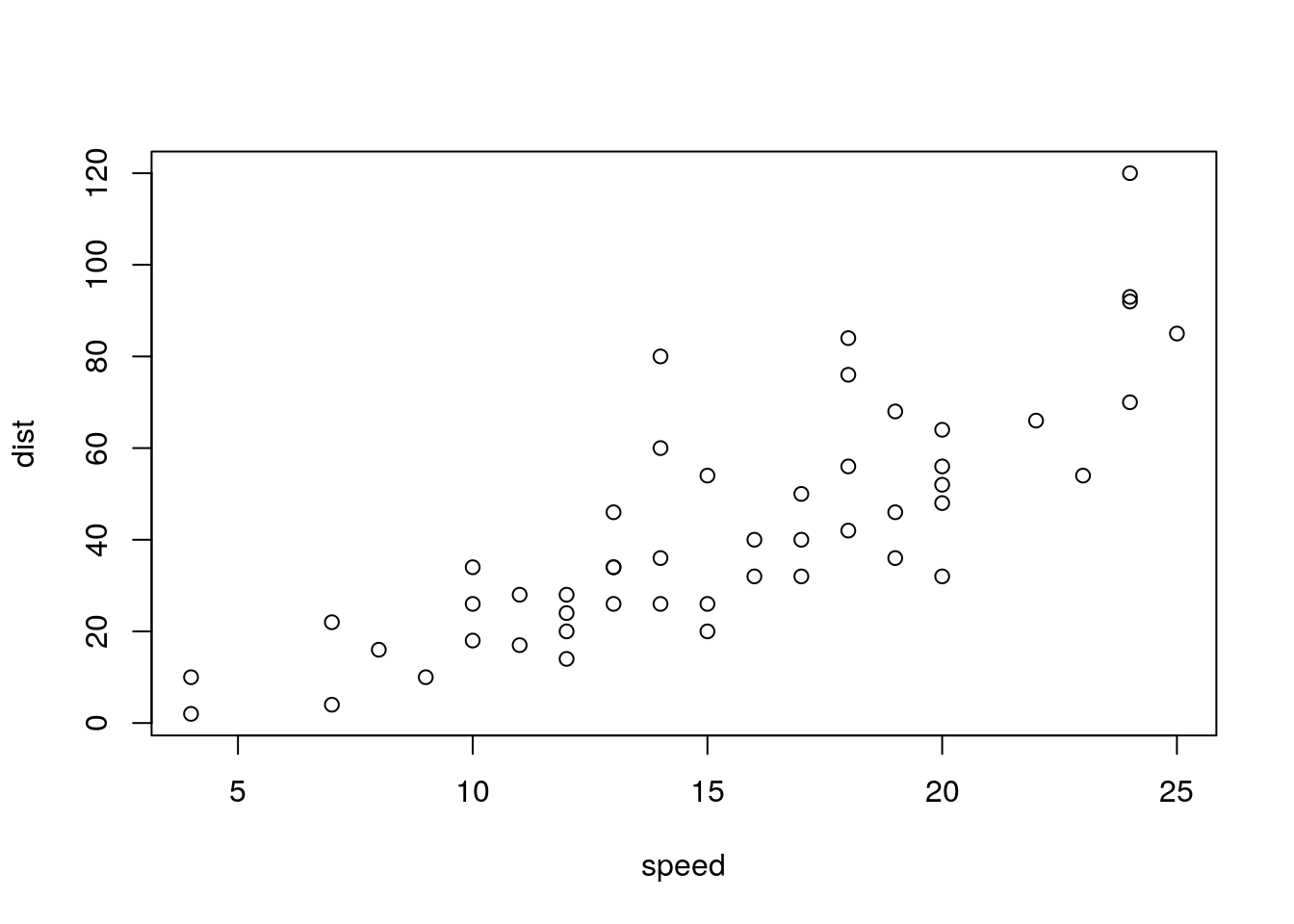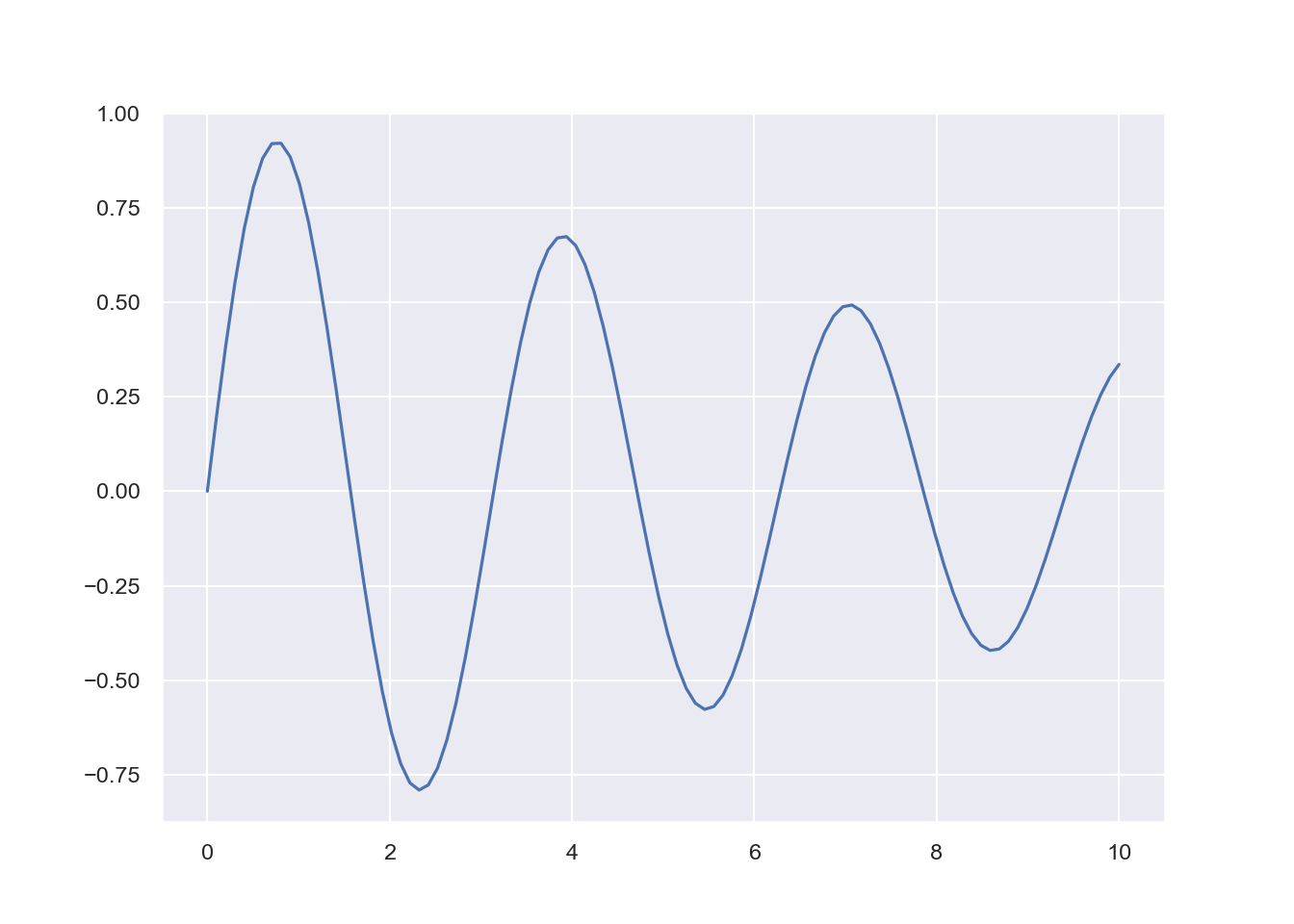CoCalc Public Filesrmd-equation-numbering / equation-numbering.html
Compute Environment: Ubuntu 18.04 (Deprecated)
(File too big to render with math typesetting.)
References in R Markdown

# 1 Introduction

Using RMarkdown’s bookdown rendering, long documents with LaTeX-style references can be rendered. More information here: bookdown: Authoring Books and Technical Documents with R Markdown

$\begin{equation} E = m c^2 \tag{1.1} \end{equation}$

# 2 Examples

## 2.1 Example 1

$\begin{equation} f\left(k\right) = \binom{n}{k} p^k\left(1-p\right)^{n-k} \tag{2.1} \end{equation}$

The equation (2.1) is well known.

## 2.2 Example 2

Another well known formula is the following:

Theorem 2.1 (Pythagorean theorem) For a right triangle, if $$c$$ denotes the length of the hypotenuse and $$a$$ and $$b$$ denote the lengths of the other two sides, we have

$\begin{equation} a^2 + b^2 = c^2 \tag{2.2} \end{equation}$

Which is this equation:

# 3 Fill up

A normal paragraph.

plot(cars)  # a scatterplotFigure 3.1: A scatterplot of the data cars using base R graphics.

More text …

More text …

## 3.1 Python code

import numpy as np
import pandas as pd
x = np.random.randn(1000)
pd.Series(x).describe()
## count    1000.000000
## mean        0.074123
## std         0.971023
## min        -3.503836
## 25%        -0.563154
## 50%         0.092345
## 75%         0.724961
## max         3.365597
## dtype: float64

### 3.1.1 Plotting with Python

import seaborn
seaborn.set('paper')
import numpy as np
import matplotlib.pyplot as plt
xx = np.linspace(0, 10, 100)
yy = np.exp(0.1 * -xx) * np.sin(2 * xx)
plt.plot(xx, yy)
plt.grid(True)
plt.show()More text …

More text …

More text …

More text …

More text …

More text …

More text …

More text …

More text …

More text …

More text …